# 半空间中具有非理想界面的圆柱形芯–壳结构对P波的多重衍射Multiple Diffraction of P Wave by Cylindrical Core-Shell Structure with Non-Ideal Interface in Half Space

DOI: 10.12677/IJM.2020.92006, PDF, HTML, XML, 下载: 42  浏览: 69  国家自然科学基金支持

Abstract: In this paper, the wave function expansion method is used to analyze the multiple diffraction of plane P wave by cylindrical core-shell structure with non-ideal interface in half space, and the an-alytical solution of dynamic stress concentration factor is obtained. A spring model is used to sim-ulate the non-ideal interface. By changing the material parameters, spring coefficient and incident angle, the variation of dynamic stress concentration factor at different incident wave frequencies is discussed. The results show that the surface parameters have a significant effect on the dynamic stress concentration at the interface at the nanometer scale. The dynamic stress concentration factor at the interface is close to that at the ideal interface by increasing the elastic coefficient of the spring. When the frequency and angle of incident wave are changed, the dynamic stress con-centration factor is obviously different. Using different spring models to simulate the imperfect interface for different practical problems can make the research results more accurate.

1. 研究背景

2. 模型与公式

2.1. 模型建立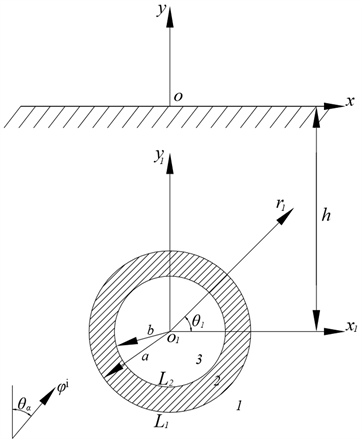Figure 1. Multiple diffraction of P wave by cylindrical core-shell structure with non-ideal interface in half space

2.2. 波函数在不同坐标系下的基本方程

${\phi }^{\text{i}}\left(x,y\right)={\phi }_{0}\mathrm{exp}\left[\text{I}{k}_{\alpha 1}\left(x\mathrm{sin}{\theta }_{\alpha }+y\mathrm{cos}{\theta }_{\alpha }\right)-\text{I}\omega \text{ }t\right]$ (1)

${\phi }^{\text{r}}\left(x,y\right)={K}_{1}\mathrm{exp}\left[\text{I}{k}_{\alpha 1}\left(x\mathrm{sin}{\theta }_{\alpha }-y\mathrm{cos}{\theta }_{\alpha }\right)-\text{I}\omega t\right]$ (2)

${\psi }^{\text{r}}\left(x,y\right)={K}_{1}\mathrm{exp}\left[\text{I}{k}_{\beta 1}\left(x\mathrm{sin}{\theta }_{\beta }-y\mathrm{cos}{\theta }_{\beta }\right)-\text{I}\omega t\right]$ (3)

${\phi }^{\text{i}}\left({r}_{1},{\theta }_{1}\right)=\underset{n=-\infty }{\overset{+\infty }{\sum }}{J}_{n}\left({k}_{\alpha 1}{r}_{1}\right)\mathrm{exp}\left(\text{I}n{\theta }_{\alpha }+\text{I}n{\theta }_{1}-\text{I}{k}_{\alpha 1}h\mathrm{cos}{\theta }_{\alpha }\right)$ (4)

${\phi }^{\text{r}}\left({r}_{1},{\theta }_{1}\right)=\underset{n=-\infty }{\overset{+\infty }{\sum }}{\left(-1\right)}^{n}{K}_{1}{J}_{n}\left({k}_{\alpha 1}{r}_{1}\right)\mathrm{exp}\left(-\text{I}n{\theta }_{\alpha }+\text{I}n{\theta }_{1}+\text{I}{k}_{\alpha 1}h\mathrm{cos}{\theta }_{\alpha }\right)$ (5)

${\psi }^{\text{r}}\left({r}_{1},{\theta }_{1}\right)=\underset{n=-\infty }{\overset{+\infty }{\sum }}{\left(-1\right)}^{n}{K}_{2}{J}_{n}\left({k}_{\beta 1}{r}_{1}\right)\mathrm{exp}\left(-\text{I}n{\theta }_{\beta }+\text{I}n{\theta }_{1}+\text{I}{k}_{\beta 1}h\mathrm{cos}{\theta }_{\beta }\right)$ (6)

${\phi }^{\text{s}}\left({r}_{1},{\theta }_{1}\right)=\underset{n=-\infty }{\overset{+\infty }{\sum }}{A}_{1n}{H}_{n}^{\left(1\right)}\left({k}_{\alpha 1}{r}_{1}\right)\mathrm{exp}\left(\text{I}n{\theta }_{1}\right)$ (7)

${\psi }^{\text{s}}\left({r}_{1},{\theta }_{1}\right)=\underset{n=-\infty }{\overset{+\infty }{\sum }}{B}_{1n}{H}_{n}^{\left(1\right)}\left({k}_{\beta 1}{r}_{1}\right)\mathrm{exp}\left(\text{I}n{\theta }_{1}\right)$ (8)

$\begin{array}{c}{\phi }^{\left(1\right)}\left({r}_{1},{\theta }_{1}\right)={\phi }^{\text{i}}\left({r}_{1},{\theta }_{1}\right)+{\phi }^{\text{r}}\left({r}_{1},{\theta }_{1}\right)+{\phi }^{\text{s}}\left({r}_{1},{\theta }_{1}\right)\\ =\underset{n=-\infty }{\overset{+\infty }{\sum }}\left[{A}_{0n}{J}_{n}\left({k}_{\alpha 1}{r}_{1}\right)+{A}_{1n}{H}_{n}^{\left(1\right)}\left({k}_{\alpha 1}{r}_{1}\right)\right]\mathrm{exp}\left(\text{I}n{\theta }_{1}\right)\end{array}$ (9)

$\begin{array}{c}{\psi }^{\left(1\right)}\left({r}_{1},{\theta }_{1}\right)={\psi }^{\text{r}}\left({r}_{1},{\theta }_{1}\right)+{\psi }^{\text{s}}\left({r}_{1},{\theta }_{1}\right)\\ =\underset{n=-\infty }{\overset{+\infty }{\sum }}\left[{B}_{0n}{J}_{n}\left({k}_{\beta 1}{r}_{1}\right)+{B}_{1n}{H}_{n}^{\left(1\right)}\left({k}_{\beta 1}{r}_{1}\right)\right]\mathrm{exp}\left(\text{I}n{\theta }_{1}\right)\end{array}$ (10)

${\phi }^{\left(2\right)}\left({r}_{1},{\theta }_{1}\right)=\underset{n=-\infty }{\overset{+\infty }{\sum }}\left[{A}_{2n}{J}_{n}\left({k}_{\alpha 2}{r}_{1}\right)+{C}_{2n}{H}_{n}^{\left(2\right)}\left({k}_{\alpha 2}{r}_{1}\right)\right]\mathrm{exp}\left(\text{I}n{\theta }_{1}\right)$ (11)

${\psi }^{\left(2\right)}\left({r}_{1},{\theta }_{1}\right)=\underset{n=-\infty }{\overset{+\infty }{\sum }}\left[{B}_{2n}{J}_{n}\left({k}_{\beta 2}{r}_{1}\right)+{D}_{2n}{H}_{n}^{\left(2\right)}\left({k}_{\beta 2}{r}_{1}\right)\right]\mathrm{exp}\left(\text{I}n{\theta }_{1}\right)$ (12)

${\phi }^{\left(3\right)}\left({r}_{1},{\theta }_{1}\right)=\underset{n=-\infty }{\overset{+\infty }{\sum }}{A}_{3n}{J}_{n}\left({k}_{\alpha 3}{r}_{1}\right)\mathrm{exp}\left(\text{I}n{\theta }_{1}\right)$ (13)

${\psi }^{\left(3\right)}\left({r}_{1},{\theta }_{1}\right)=\underset{n=-\infty }{\overset{+\infty }{\sum }}{B}_{3n}{J}_{n}\left({k}_{\beta 3}{r}_{1}\right)\mathrm{exp}\left(\text{I}n{\theta }_{1}\right)$ (14)

${u}_{r}=\frac{\partial \phi }{\partial r}+\frac{1}{r}\frac{\partial \psi }{\partial \theta }$ (15)

${u}_{\theta }=\frac{1}{r}\frac{\partial \phi }{\partial \theta }-\frac{\partial \psi }{\partial r}$ (16)

${\sigma }_{rr}=\lambda \left(\frac{{\partial }^{2}\phi }{\partial {r}^{2}}+\frac{1}{r}\frac{\partial \phi }{\partial r}+\frac{1}{{r}^{2}}\frac{{\partial }^{2}\phi }{\partial {\theta }^{2}}\right)+2\mu \left(\frac{{\partial }^{2}\phi }{\partial {r}^{2}}-\frac{1}{{r}^{2}}\frac{\partial \psi }{\partial \theta }+\frac{1}{r}\frac{{\partial }^{2}\psi }{\partial r\partial \theta }\right)$ (17)

${\sigma }_{\theta \theta }=\lambda \left(\frac{{\partial }^{2}\phi }{\partial {r}^{2}}+\frac{1}{r}\frac{\partial \phi }{\partial r}+\frac{1}{{r}^{2}}\frac{{\partial }^{2}\phi }{\partial {\theta }^{2}}\right)+2\mu \left(\frac{1}{{r}^{2}}\frac{{\partial }^{2}\phi }{\partial {\theta }^{2}}+\frac{1}{r}\frac{\partial \phi }{\partial r}-\frac{1}{r}\frac{{\partial }^{2}\psi }{\partial r\partial \theta }+\frac{1}{{r}^{2}}\frac{\partial \psi }{\partial \theta }\right)$ (18)

${\sigma }_{r\theta }=\frac{\mu }{r}\left(2\frac{{\partial }^{2}\phi }{\partial r\partial \theta }-\frac{2}{r}\frac{\partial \phi }{\partial \theta }+\frac{1}{r}\frac{{\partial }^{2}\psi }{\partial {\theta }^{2}}-r\frac{{\partial }^{2}\psi }{\partial {r}^{2}}+\frac{\partial \psi }{\partial r}\right)$ (19)

${u}_{{r}_{1}}^{\left(1\right)}=\frac{1}{{r}_{1}}\underset{n=-\infty }{\overset{\infty }{\sum }}\left[{A}_{0n}{M}_{71}^{\left(1\right)}\left({k}_{\alpha 1}{r}_{1}\right)+{A}_{1n}{M}_{71}^{\left(3\right)}\left({k}_{\alpha 1}{r}_{1}\right)+\text{I}{B}_{0n}{M}_{72}^{\left(1\right)}\left({k}_{\beta 1}{r}_{1}\right)+\text{I}{B}_{1n}{M}_{72}^{\left(3\right)}\left({k}_{\beta 1}{r}_{1}\right)\right]\mathrm{exp}\left(\text{I}n{\theta }_{1}\right)$ (20)

${u}_{{\theta }_{1}}^{\left(1\right)}=\frac{1}{{r}_{1}}\underset{n=-\infty }{\overset{\infty }{\sum }}\left[\text{I}{A}_{0n}{M}_{81}^{\left(1\right)}\left({k}_{\alpha 1}{r}_{1}\right)+\text{I}{A}_{1n}{M}_{81}^{\left(3\right)}\left({k}_{\alpha 1}{r}_{1}\right)+{B}_{0n}{M}_{82}^{\left(1\right)}\left({k}_{\beta 1}{r}_{1}\right)+{B}_{1n}{M}_{82}^{\left(3\right)}\left({k}_{\beta 1}{r}_{1}\right)\right]\mathrm{exp}\left(\text{I}n{\theta }_{1}\right)$ (21)

${\sigma }_{{r}_{1}{r}_{1}}^{\left(1\right)}=\frac{2{\mu }^{\left(1\right)}}{{r}_{1}^{2}}\underset{n=-\infty }{\overset{\infty }{\sum }}\left[{A}_{0n}{E}_{11}^{\left(1\right)}\left({k}_{\alpha 1}{r}_{1}\right)+{A}_{1n}{E}_{11}^{\left(3\right)}\left({k}_{\alpha 1}{r}_{1}\right)+\text{I}{B}_{0n}{E}_{12}^{\left(1\right)}\left({k}_{\beta 1}{r}_{1}\right)+\text{I}{B}_{1n}{E}_{12}^{\left(3\right)}\left({k}_{\beta 1}{r}_{1}\right)\right]\mathrm{exp}\left(\text{I}n{\theta }_{1}\right)$ (22)

${\sigma }_{{\theta }_{1}{\theta }_{1}}^{\left(1\right)}=\frac{2{\mu }^{\left(1\right)}}{{r}_{1}^{2}}\underset{n=-\infty }{\overset{\infty }{\sum }}\left[{A}_{0n}{E}_{21}^{\left(1\right)}\left({k}_{\alpha 1}{r}_{1}\right)+{A}_{1n}{E}_{21}^{\left(3\right)}\left({k}_{\alpha 1}{r}_{1}\right)+\text{I}{B}_{0n}{E}_{22}^{\left(1\right)}\left({k}_{\beta 1}{r}_{1}\right)+\text{I}{B}_{1n}{E}_{22}^{\left(3\right)}\left({k}_{\beta 1}{r}_{1}\right)\right]\mathrm{exp}\left(\text{I}n{\theta }_{1}\right)$ (23)

${\sigma }_{{r}_{1}{\theta }_{1}}^{\left(1\right)}=\frac{2{\mu }^{\left(1\right)}}{{r}_{1}^{2}}\underset{n=-\infty }{\overset{\infty }{\sum }}\left[\text{I}{A}_{0n}{E}_{41}^{\left(1\right)}\left({k}_{\alpha 1}{r}_{1}\right)+\text{I}{A}_{1n}{E}_{41}^{\left(3\right)}\left({k}_{\alpha 1}{r}_{1}\right)+{B}_{0n}{E}_{42}^{\left(1\right)}\left({k}_{\beta 1}{r}_{1}\right)+{B}_{1n}{E}_{42}^{\left(3\right)}\left({k}_{\beta 1}{r}_{1}\right)\right]\mathrm{exp}\left(\text{I}n{\theta }_{1}\right)$ (24)

${u}_{{r}_{1}}^{\left(2\right)}=\frac{1}{{r}_{1}}\underset{n=-\infty }{\overset{\infty }{\sum }}\left[{A}_{2n}{M}_{71}^{\left(1\right)}\left({k}_{\alpha 2}{r}_{1}\right)+{C}_{2n}{M}_{71}^{\left(4\right)}\left({k}_{\alpha 2}{r}_{1}\right)+\text{I}{B}_{2n}{M}_{72}^{\left(1\right)}\left({k}_{\beta 2}{r}_{1}\right)+\text{I}{D}_{2n}{M}_{72}^{\left(4\right)}\left({k}_{\beta 2}{r}_{1}\right)\right]\mathrm{exp}\left(\text{I}n{\theta }_{1}\right)$ (25)

${u}_{{\theta }_{1}}^{\left(2\right)}=\frac{1}{{r}_{1}}\underset{n=-\infty }{\overset{\infty }{\sum }}\left[\text{I}{A}_{2n}{M}_{81}^{\left(1\right)}\left({k}_{\alpha 2}{r}_{1}\right)+\text{I}{C}_{2n}{M}_{81}^{\left(4\right)}\left({k}_{\alpha 2}{r}_{1}\right)+{B}_{2n}{M}_{82}^{\left(1\right)}\left({k}_{\beta 2}{r}_{1}\right)+{D}_{2n}{M}_{82}^{\left(4\right)}\left({k}_{\beta 2}{r}_{1}\right)\right]\mathrm{exp}\left(\text{I}n{\theta }_{1}\right)$ (26)

${\sigma }_{{r}_{1}{r}_{1}}^{\left(2\right)}=\frac{2{\mu }^{\left(2\right)}}{{r}_{1}^{2}}\underset{n=-\infty }{\overset{\infty }{\sum }}\left[{A}_{2n}{E}_{11}^{\left(1\right)}\left({k}_{\alpha 2}{r}_{1}\right)+{C}_{2n}{E}_{11}^{\left(4\right)}\left({k}_{\alpha 2}{r}_{1}\right)+\text{I}{B}_{2n}{E}_{12}^{\left(1\right)}\left({k}_{\beta 2}{r}_{1}\right)+\text{I}{D}_{2n}{E}_{12}^{\left(4\right)}\left({k}_{\beta 2}{r}_{1}\right)\right]\mathrm{exp}\left(\text{I}n{\theta }_{1}\right)$ (27)

${\sigma }_{{\theta }_{1}{\theta }_{1}}^{\left(2\right)}=\frac{2{\mu }^{\left(2\right)}}{{r}_{1}^{2}}\underset{n=-\infty }{\overset{\infty }{\sum }}\left[{A}_{2n}{E}_{21}^{\left(1\right)}\left({k}_{\alpha 2}{r}_{1}\right)+{C}_{2n}{E}_{21}^{\left(4\right)}\left({k}_{\alpha 2}{r}_{1}\right)+\text{I}{B}_{2n}{E}_{22}^{\left(1\right)}\left({k}_{\beta 2}{r}_{1}\right)+\text{I}{D}_{2n}{E}_{22}^{\left(4\right)}\left({k}_{\beta 2}{r}_{1}\right)\right]\mathrm{exp}\left(\text{I}n{\theta }_{1}\right)$ (28)

${\sigma }_{{r}_{1}{\theta }_{1}}^{\left(2\right)}=\frac{2{\mu }^{\left(2\right)}}{{r}_{1}^{2}}\underset{n=-\infty }{\overset{\infty }{\sum }}\left[\text{I}{A}_{2n}{E}_{41}^{\left(1\right)}\left({k}_{\alpha 2}{r}_{1}\right)+\text{I}{C}_{2n}{E}_{41}^{\left(4\right)}\left({k}_{\alpha 2}{r}_{1}\right)+{B}_{2n}{E}_{42}^{\left(1\right)}\left({k}_{\beta 2}{r}_{1}\right)+{D}_{2n}{E}_{42}^{\left(4\right)}\left({k}_{\beta 2}{r}_{1}\right)\right]\mathrm{exp}\left(\text{I}n{\theta }_{1}\right)$ (29)

${u}_{{r}_{1}}^{\left(3\right)}=\frac{1}{{r}_{1}}\underset{n=-\infty }{\overset{\infty }{\sum }}\left[{A}_{3n}{M}_{71}^{\left(1\right)}\left({k}_{\alpha 3}{r}_{1}\right)+\text{I}{B}_{3n}{M}_{72}^{\left(1\right)}\left({k}_{\beta 3}{r}_{1}\right)\right]\mathrm{exp}\left(\text{I}n{\theta }_{1}\right)$ (30)

${u}_{{\theta }_{1}}^{\left(3\right)}=\frac{1}{{r}_{1}}\underset{n=-\infty }{\overset{\infty }{\sum }}\left[\text{I}{A}_{3n}{M}_{81}^{\left(1\right)}\left({k}_{\alpha 3}{r}_{1}\right)+{B}_{3n}{M}_{82}^{\left(1\right)}\left({k}_{\beta 3}{r}_{1}\right)\right]\mathrm{exp}\left(\text{I}n{\theta }_{1}\right)$ (31)

${\sigma }_{{r}_{1}{r}_{1}}^{\left(3\right)}=\frac{2{\mu }^{\left(3\right)}}{{r}_{1}^{2}}\underset{n=-\infty }{\overset{\infty }{\sum }}\left[{A}_{3n}{E}_{11}^{\left(1\right)}\left({k}_{\alpha 3}{r}_{1}\right)+\text{I}{B}_{3n}{E}_{12}^{\left(1\right)}\left({k}_{\beta 3}{r}_{1}\right)\right]\mathrm{exp}\left(\text{I}n{\theta }_{1}\right)$ (32)

${\sigma }_{{\theta }_{1}{\theta }_{1}}^{\left(3\right)}=\frac{2{\mu }^{\left(3\right)}}{{r}_{1}^{2}}\underset{n=-\infty }{\overset{\infty }{\sum }}\left[{A}_{3n}{E}_{21}^{\left(1\right)}\left({k}_{\alpha 3}{r}_{1}\right)+\text{I}{B}_{3n}{E}_{22}^{\left(1\right)}\left({k}_{\beta 3}{r}_{1}\right)\right]\mathrm{exp}\left(\text{I}n{\theta }_{1}\right)$ (33)

${\sigma }_{{r}_{1}{\theta }_{1}}^{\left(3\right)}=\frac{2{\mu }^{\left(3\right)}}{{r}_{1}^{2}}\underset{n=-\infty }{\overset{\infty }{\sum }}\left[\text{I}{A}_{3n}{E}_{41}^{\left(1\right)}\left({k}_{\alpha 3}{r}_{1}\right)+{B}_{3n}{E}_{42}^{\left(1\right)}\left({k}_{\beta 3}{r}_{1}\right)\right]\mathrm{exp}\left(\text{I}n{\theta }_{1}\right)$ (34)

2.3. 边界条件

2.3.1. 位移边界条件

${u}_{{r}_{1}}^{\left(1\right)}-{u}_{{r}_{1}}^{\left(2\right)}=\frac{{\sigma }_{{r}_{1}{r}_{1}}^{\left(1\right)}}{{k}_{{r}_{1}}^{\left(1\right)}},{u}_{{\theta }_{1}}^{\left(1\right)}-{u}_{{\theta }_{1}}^{\left(2\right)}=\frac{{\sigma }_{{r}_{1}{\theta }_{1}}^{\left(1\right)}}{{k}_{{\theta }_{1}}^{\left(1\right)}},{r}_{1}=a$ (35)

${u}_{{r}_{1}}^{\left(3\right)}-{u}_{{r}_{1}}^{\left(2\right)}=\frac{{\sigma }_{{r}_{1}{r}_{1}}^{\left(3\right)}}{{k}_{{r}_{1}}^{\left(3\right)}},{u}_{{\theta }_{1}}^{\left(3\right)}-{u}_{{\theta }_{1}}^{\left(2\right)}=\frac{{\sigma }_{{r}_{1}{\theta }_{1}}^{\left(3\right)}}{{k}_{{\theta }_{1}}^{\left(3\right)}},{r}_{1}=b$ (36)

2.3.2. 应力边界条件

$\left\{\begin{array}{l}{\sigma }_{{r}_{1}{r}_{1}}^{\left(1\right)}-{\sigma }_{{r}_{1}{r}_{1}}^{\left(2\right)}={s}_{1}\left(1-{\nu }^{\left(1\right)}\right){\sigma }_{{\theta }_{1}{\theta }_{1}}^{\left(1\right)}-{s}_{1}{\nu }^{\left(1\right)}{\sigma }_{{r}_{1}{r}_{1}}^{\left(1\right)}\\ {\sigma }_{{r}_{1}{\theta }_{1}}^{\left(1\right)}-{\sigma }_{{r}_{1}{\theta }_{1}}^{\left(2\right)}=-{s}_{1}\left(1-{\nu }^{\left(1\right)}\right)\frac{\partial {\sigma }_{{\theta }_{1}{\theta }_{1}}^{\left(1\right)}}{\partial {\theta }_{1}}+{s}_{1}{\nu }^{\left(1\right)}\frac{\partial {\sigma }_{{r}_{1}{r}_{1}}^{\left(1\right)}}{\partial {\theta }_{1}}\end{array},{r}_{1}=a$ (37)

$\left\{\begin{array}{l}{\sigma }_{{r}_{1}{r}_{1}}^{\left(3\right)}-{\sigma }_{{r}_{1}{r}_{1}}^{\left(2\right)}={s}_{2}\left(1-{\nu }^{\left(3\right)}\right){\sigma }_{{\theta }_{1}{\theta }_{1}}^{\left(3\right)}-{s}_{2}{\nu }^{\left(3\right)}{\sigma }_{{r}_{1}{r}_{1}}^{\left(3\right)}\\ {\sigma }_{{r}_{1}{\theta }_{1}}^{\left(3\right)}-{\sigma }_{{r}_{1}{\theta }_{1}}^{\left(2\right)}=-{s}_{2}\left(1-{\nu }^{\left(3\right)}\right)\frac{\partial {\sigma }_{{\theta }_{1}{\theta }_{1}}^{\left(3\right)}}{\partial {\theta }_{1}}+{s}_{2}{\nu }^{\left(3\right)}\frac{\partial {\sigma }_{{r}_{1}{r}_{1}}^{\left(3\right)}}{\partial {\theta }_{1}}\end{array},{r}_{1}=b$ (38)

${s}_{1}=\frac{2{\mu }^{s}+{\lambda }^{s}}{2{\mu }^{\left(2\right)}a},{s}_{2}=\frac{2{\mu }^{s}+{\lambda }^{s}}{2{\mu }^{\left(2\right)}b}=\eta {s}_{1},\eta =\frac{a}{b}$ (39)

$\begin{array}{l}{A}_{0n}{M}_{71}^{\left(1\right)}\left({k}_{\alpha 1}a\right)+{A}_{1n}{M}_{71}^{\left(3\right)}\left({k}_{\alpha 1}a\right)+\text{I}{B}_{0n}{M}_{72}^{\left(1\right)}\left({k}_{\beta 1}a\right)+\text{I}{B}_{1n}{M}_{72}^{\left(3\right)}\left({k}_{\beta 1}a\right)\\ -\left({A}_{2n}{M}_{71}^{\left(1\right)}\left({k}_{\alpha 2}a\right)+{C}_{2n}{M}_{71}^{\left(4\right)}\left({k}_{\alpha 2}a\right)+\text{I}{B}_{2n}{M}_{72}^{\left(1\right)}\left({k}_{\beta 2}a\right)+\text{I}{D}_{2n}{M}_{72}^{\left(4\right)}\left({k}_{\beta 2}a\right)\right)\\ =\frac{2{\mu }^{\left(1\right)}}{{k}_{{r}_{1}}^{\left(1\right)}a}\left({A}_{0n}{E}_{11}^{\left(1\right)}\left({k}_{\alpha 1}a\right)+{A}_{1n}{E}_{11}^{\left(3\right)}\left({k}_{\alpha 1}a\right)+\text{I}{B}_{0n}{E}_{12}^{\left(1\right)}\left({k}_{\beta 1}a\right)+\text{I}{B}_{1n}{E}_{12}^{\left(3\right)}\left({k}_{\beta 1}a\right)\right)\end{array}$ (40)

$\begin{array}{l}\text{I}{A}_{0n}{M}_{81}^{\left(1\right)}\left({k}_{\alpha 1}a\right)+\text{I}{A}_{1n}{M}_{81}^{\left(3\right)}\left({k}_{\alpha 1}a\right)+{B}_{0n}{M}_{82}^{\left(1\right)}\left({k}_{\beta 1}a\right)+{B}_{1n}{M}_{82}^{\left(3\right)}\left({k}_{\beta 1}a\right)\\ -\left(\text{I}{A}_{2n}{M}_{81}^{\left(1\right)}\left({k}_{\alpha 2}a\right)+\text{I}{C}_{2n}{M}_{81}^{\left(4\right)}\left({k}_{\alpha 2}a\right)+{B}_{2n}{M}_{82}^{\left(1\right)}\left({k}_{\beta 2}a\right)+{D}_{2n}{M}_{82}^{\left(4\right)}\left({k}_{\beta 2}a\right)\right)\\ =\frac{2{\mu }^{\left(1\right)}}{{k}_{{\theta }_{1}}^{\left(1\right)}a}\left(\text{I}{A}_{0n}{E}_{41}^{\left(1\right)}\left({k}_{\alpha 1}a\right)+\text{I}{A}_{1n}{E}_{41}^{\left(3\right)}\left({k}_{\alpha 1}a\right)+{B}_{0n}{E}_{42}^{\left(1\right)}\left({k}_{\beta 1}a\right)+{B}_{1n}{E}_{42}^{\left(3\right)}\left({k}_{\beta 1}a\right)\right)\end{array}$ (41)

$\begin{array}{l}\text{I}{A}_{3n}{M}_{81}^{\left(1\right)}\left({k}_{\alpha 3}b\right)+{B}_{3n}{M}_{82}^{\left(1\right)}\left({k}_{\beta 3}b\right)\\ -\left(\text{I}{A}_{2n}{M}_{81}^{\left(1\right)}\left({k}_{\alpha 2}b\right)+\text{I}{C}_{2n}{M}_{81}^{\left(4\right)}\left({k}_{\alpha 2}b\right)+{B}_{2n}{M}_{82}^{\left(1\right)}\left({k}_{\beta 2}b\right)+{D}_{2n}{M}_{82}^{\left(4\right)}\left({k}_{\beta 2}b\right)\right)\\ =\frac{2{\mu }^{\left(3\right)}}{{k}_{{\theta }_{1}}^{\left(3\right)}b}\left(\text{I}{A}_{3n}{E}_{41}^{\left(1\right)}\left({k}_{\alpha 3}b\right)+{B}_{3n}{E}_{42}^{\left(1\right)}\left({k}_{\beta 3}b\right)\right)\end{array}$ (42)

$\begin{array}{l}\text{I}{A}_{3n}{M}_{81}^{\left(1\right)}\left({k}_{\alpha 3}b\right)+{B}_{3n}{M}_{82}^{\left(1\right)}\left({k}_{\beta 3}b\right)\\ -\left(\text{I}{A}_{2n}{M}_{81}^{\left(1\right)}\left({k}_{\alpha 2}b\right)+\text{I}{C}_{2n}{M}_{81}^{\left(4\right)}\left({k}_{\alpha 2}b\right)+{B}_{2n}{M}_{82}^{\left(1\right)}\left({k}_{\beta 2}b\right)+{D}_{2n}{M}_{82}^{\left(4\right)}\left({k}_{\beta 2}b\right)\right)\\ =\frac{2{\mu }^{\left(3\right)}}{{k}_{{\theta }_{1}}^{\left(3\right)}b}\left(\text{I}{A}_{3n}{E}_{41}^{\left(1\right)}\left({k}_{\alpha 3}b\right)+{B}_{3n}{E}_{42}^{\left(1\right)}\left({k}_{\beta 3}b\right)\right)\end{array}$ (43)

$\begin{array}{l}\left({A}_{0n}{E}_{11}^{\left(1\right)}\left({k}_{\alpha 1}a\right)+{A}_{1n}{E}_{11}^{\left(3\right)}\left({k}_{\alpha 1}a\right)+\text{I}{B}_{0n}{E}_{12}^{\left(1\right)}\left({k}_{\beta 1}a\right)+\text{I}{B}_{1n}{E}_{12}^{\left(3\right)}\left({k}_{\beta 1}a\right)\right)\\ -\frac{{\mu }^{\left(2\right)}}{{\mu }^{\left(1\right)}}\left({A}_{2n}{E}_{11}^{\left(1\right)}\left({k}_{\alpha 2}a\right)+{C}_{2n}{E}_{11}^{\left(4\right)}\left({k}_{\alpha 2}a\right)+\text{I}{B}_{2n}{E}_{12}^{\left(1\right)}\left({k}_{\beta 2}a\right)+\text{I}{D}_{2n}{E}_{12}^{\left(4\right)}\left({k}_{\beta 2}a\right)\right)\\ ={s}_{\text{1}}\left(1-{\nu }^{\left(1\right)}\right)\left({A}_{0n}{E}_{21}^{\left(1\right)}\left({k}_{\alpha 1}a\right)+{A}_{1n}{E}_{21}^{\left(3\right)}\left({k}_{\alpha 1}a\right)+\text{I}{B}_{0n}{E}_{22}^{\left(1\right)}\left({k}_{\beta 1}a\right)+\text{I}{B}_{1n}{E}_{22}^{\left(3\right)}\left({k}_{\beta 1}a\right)\right)\\ \text{ }\text{ }\text{ }\text{ }\text{ }\text{ }\text{ }\text{ }-{s}_{\text{1}}{\nu }^{\left(1\right)}\left({A}_{0n}{E}_{11}^{\left(1\right)}\left({k}_{\alpha 1}a\right)+{A}_{1n}{E}_{11}^{\left(3\right)}\left({k}_{\alpha 1}a\right)+\text{I}{B}_{0n}{E}_{12}^{\left(1\right)}\left({k}_{\beta 1}a\right)+\text{I}{B}_{1n}{E}_{12}^{\left(3\right)}\left({k}_{\beta 1}a\right)\right)\end{array}$ (44)

$\begin{array}{l}\left(\text{I}{A}_{0n}{E}_{41}^{\left(1\right)}\left({k}_{\alpha 1}a\right)+\text{I}{A}_{1n}{E}_{41}^{\left(3\right)}\left({k}_{\alpha 1}a\right)+{B}_{0n}{E}_{42}^{\left(1\right)}\left({k}_{\beta 1}a\right)+{B}_{1n}{E}_{42}^{\left(3\right)}\left({k}_{\beta 1}a\right)\right)\\ -\frac{{\mu }^{\left(2\right)}}{{\mu }^{\left(1\right)}}\left(\text{I}{A}_{2n}{E}_{41}^{\left(1\right)}\left({k}_{\alpha 2}a\right)+\text{I}{C}_{2n}{E}_{41}^{\left(4\right)}\left({k}_{\alpha 2}a\right)+{B}_{2n}{E}_{42}^{\left(1\right)}\left({k}_{\beta 2}a\right)+{D}_{2n}{E}_{42}^{\left(4\right)}\left({k}_{\beta 2}a\right)\right)\\ =-\text{I}n{s}_{\text{1}}\left(1-{\nu }^{\left(1\right)}\right)\left(\text{I}{A}_{0n}{E}_{21}^{\left(1\right)}\left({k}_{\alpha 1}a\right)+\text{I}{A}_{1n}{E}_{21}^{\left(3\right)}\left({k}_{\alpha 1}a\right)+{B}_{0n}{E}_{22}^{\left(1\right)}\left({k}_{\beta 1}a\right)+{B}_{1n}{E}_{22}^{\left(3\right)}\left({k}_{\beta 1}a\right)\right)\\ \text{ }\text{ }\text{ }\text{ }\text{ }\text{ }\text{ }\text{ }\text{ }+\text{I}n{s}_{1}{\nu }^{\left(1\right)}\left(\text{I}{A}_{0n}{E}_{11}^{\left(1\right)}\left({k}_{\alpha 1}a\right)+\text{I}{A}_{1n}{E}_{11}^{\left(3\right)}\left({k}_{\alpha 1}a\right)+{B}_{0n}{E}_{12}^{\left(1\right)}\left({k}_{\beta 1}a\right)+{B}_{1n}{E}_{12}^{\left(3\right)}\left({k}_{\beta 1}a\right)\right)\end{array}$ (45)

$\begin{array}{l}\left({A}_{3n}{E}_{11}^{\left(1\right)}\left({k}_{\alpha 3}b\right)+\text{I}{B}_{3n}{E}_{12}^{\left(1\right)}\left({k}_{\beta 3}b\right)\right)\\ -\frac{{\mu }^{\left(2\right)}}{{\mu }^{\left(3\right)}}\left({A}_{2n}{E}_{11}^{\left(1\right)}\left({k}_{\alpha 2}b\right)+{C}_{2n}{E}_{11}^{\left(4\right)}\left({k}_{\alpha 2}b\right)+\text{I}{B}_{2n}{E}_{12}^{\left(1\right)}\left({k}_{\beta 2}b\right)+\text{I}{D}_{2n}{E}_{12}^{\left(4\right)}\left({k}_{\beta 2}b\right)\right)\\ ={s}_{\text{2}}\left(1-{\nu }^{\left(3\right)}\right)\left({A}_{3n}{E}_{21}^{\left(1\right)}\left({k}_{\alpha 3}b\right)+\text{I}{B}_{3n}{E}_{22}^{\left(1\right)}\left({k}_{\beta 3}b\right)\right)-{s}_{\text{2}}{\nu }^{\left(3\right)}\left({A}_{3n}{E}_{11}^{\left(1\right)}\left({k}_{\alpha 3}b\right)+\text{I}{B}_{3n}{E}_{12}^{\left(1\right)}\left({k}_{\beta 3}b\right)\right)\end{array}$ (46)

$\begin{array}{l}\left(\text{I}{A}_{3n}{E}_{41}^{\left(1\right)}\left({k}_{\alpha 3}b\right)+{B}_{3n}{E}_{42}^{\left(1\right)}\left({k}_{\beta 3}b\right)\right)\\ -\frac{{\mu }^{\left(2\right)}}{{\mu }^{\left(3\right)}}\left(\text{I}{A}_{2n}{E}_{41}^{\left(1\right)}\left({k}_{\alpha 2}b\right)+\text{I}{C}_{2n}{E}_{41}^{\left(4\right)}\left({k}_{\alpha 2}b\right)+{B}_{2n}{E}_{42}^{\left(1\right)}\left({k}_{\beta 2}b\right)+{D}_{2n}{E}_{42}^{\left(4\right)}\left({k}_{\beta 2}b\right)\right)\\ =-\text{I}n{s}_{\text{2}}\left(1-{\nu }^{\left(3\right)}\right)\left(\text{I}{A}_{3n}{E}_{21}^{\left(1\right)}\left({k}_{\alpha 3}b\right)+{B}_{3n}{E}_{22}^{\left(1\right)}\left({k}_{\beta 3}b\right)\right)+\text{I}n{s}_{2}{\nu }^{\left(3\right)}\left(\text{I}{A}_{3n}{E}_{11}^{\left(1\right)}\left({k}_{\alpha 3}b\right)+{B}_{3n}{E}_{12}^{\left(1\right)}\left({k}_{\beta 3}b\right)\right)\end{array}$ (47)

2.4. 数值结果与分析

$\text{DSCF}=|\frac{{\sigma }_{{\theta }_{1}{\theta }_{1}}^{\left(1\right)}}{{\sigma }_{0}}|$ (48)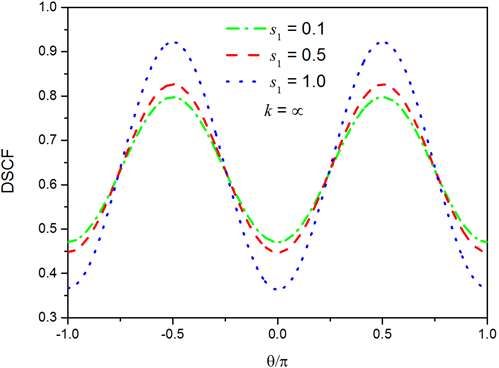Figure 2. Influence of surface parameter ${s}_{1}$ on DSCF, when ${k}_{\alpha 1}a=0.2$ and ${\theta }_{\alpha }=0.1$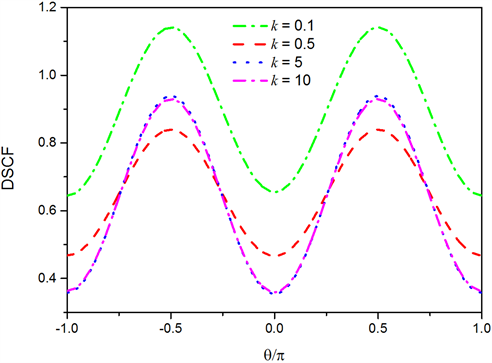Figure 3. Influence of spring coefficient k on DSCF, when ${k}_{\alpha 1}a=0.2$ and ${\theta }_{\alpha }=1.5$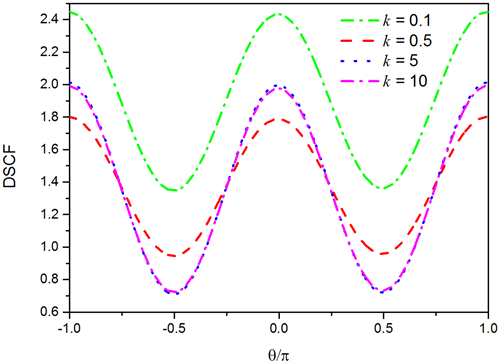Figure 4. Influence of spring coefficient k on DSCF, when ${k}_{\alpha 1}a=0.2$ and ${\theta }_{\alpha }=0.1$

3. 结论Figure 5. Influence of spring coefficient k on DSCF, when ${k}_{\alpha 1}a=\pi$ and ${\theta }_{\alpha }=1.5$

${M}_{71}^{\left(1\right)}\left({k}_{\alpha }{r}_{1}\right)=-n{J}_{n}\left({k}_{\alpha }{r}_{1}\right)+\alpha r{J}_{n-1}\left({k}_{\alpha }{r}_{1}\right)$ ${M}_{72}^{\left(1\right)}\left({k}_{\beta }{r}_{1}\right)=n{J}_{n}\left({k}_{\beta }{r}_{1}\right)$

${M}_{81}^{\left(1\right)}\left({k}_{\alpha }{r}_{1}\right)=n{J}_{n}\left({k}_{\alpha }{r}_{1}\right)$ ${M}_{82}^{\left(1\right)}\left({k}_{\beta }{r}_{1}\right)=n{J}_{n}\left({k}_{\beta }{r}_{1}\right)-{k}_{\beta }{r}_{1}{J}_{n-1}\left({k}_{\beta }{r}_{1}\right)$

${M}_{71}^{\left(4\right)}\left({k}_{\alpha }{r}_{1}\right)=-n{H}_{n}^{\left(2\right)}\left({k}_{\alpha }{r}_{1}\right)+{k}_{\alpha }{r}_{1}{H}_{n-1}^{\left(2\right)}\left({k}_{\alpha }{r}_{1}\right)$ ${M}_{72}^{\left(4\right)}\left({k}_{\beta }{r}_{1}\right)=n{H}_{n}^{\left(2\right)}\left({k}_{\beta }{r}_{1}\right)$

${M}_{81}^{\left(4\right)}\left({k}_{\alpha }{r}_{1}\right)=n{H}_{n}^{\left(2\right)}\left({k}_{\alpha }{r}_{1}\right)$ ${M}_{82}^{\left(4\right)}\left({k}_{\beta }{r}_{1}\right)=n{H}_{n}^{\left(2\right)}\left({k}_{\beta }{r}_{1}\right)-{k}_{\beta }{r}_{1}{H}_{n-1}^{\left(2\right)}\left({k}_{\beta }{r}_{1}\right)$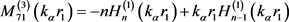${M}_{72}^{\left(3\right)}\left({k}_{\beta }{r}_{1}\right)=n{H}_{n}^{\left(1\right)}\left({k}_{\beta }{r}_{1}\right)$

${M}_{81}^{\left(3\right)}\left({k}_{\alpha }{r}_{1}\right)=n{H}_{n}^{\left(1\right)}\left({k}_{\alpha }{r}_{1}\right)$ ${M}_{82}^{\left(3\right)}\left({k}_{\beta }{r}_{1}\right)=n{H}_{n}^{\left(1\right)}\left({k}_{\beta }{r}_{1}\right)-{k}_{\beta }{r}_{1}{H}_{n-1}^{\left(1\right)}\left({k}_{\beta }{r}_{1}\right)$

${E}_{11}^{\left(1\right)}\left({k}_{\alpha }{r}_{1}\right)=\left({n}^{2}+n-\frac{{k}_{\beta }^{2}{r}_{1}^{2}}{2}\right){J}_{n}\left({k}_{\alpha }{r}_{1}\right)-{k}_{\alpha }{r}_{1}{J}_{n-1}\left({k}_{\alpha }{r}_{1}\right)$

${E}_{12}^{\left(1\right)}\left({k}_{\beta }{r}_{1}\right)=-\left({n}^{2}+n\right){J}_{n}\left({k}_{\beta }{r}_{1}\right)+n{k}_{\beta }{r}_{1}{J}_{n-1}\left({k}_{\beta }{r}_{1}\right)$

${E}_{11}^{\left(3\right)}\left({k}_{\alpha }{r}_{1}\right)=\left({n}^{2}+n-\frac{{k}_{\beta }^{2}{r}_{1}^{2}}{2}\right){H}_{n}^{\left(1\right)}\left({k}_{\alpha }{r}_{1}\right)-{k}_{\alpha }{r}_{1}{H}_{n-1}^{\left(1\right)}\left({k}_{\alpha }{r}_{1}\right)$

${E}_{12}^{\left(3\right)}\left({k}_{\beta }{r}_{1}\right)=-\left({n}^{2}+n\right){H}_{n}^{\left(1\right)}\left({k}_{\beta }{r}_{1}\right)+n{k}_{\beta }{r}_{1}{H}_{n-1}^{\left(1\right)}\left({k}_{\beta }{r}_{1}\right)$

${E}_{11}^{\left(4\right)}\left({k}_{\alpha }{r}_{1}\right)=\left({n}^{2}+n-\frac{{k}_{\beta }^{2}{r}_{1}^{2}}{2}\right){H}_{n}^{\left(2\right)}\left({k}_{\alpha }{r}_{1}\right)-{k}_{\alpha }{r}_{1}{H}_{n-1}^{\left(2\right)}\left({k}_{\alpha }{r}_{1}\right)$

${E}_{12}^{\left(4\right)}\left({k}_{\beta }{r}_{1}\right)=-\left({n}^{2}+n\right){H}_{n}^{\left(2\right)}\left({k}_{\beta }{r}_{1}\right)+n{k}_{\beta }{r}_{1}{H}_{n-1}^{\left(2\right)}\left({k}_{\beta }{r}_{1}\right)$

${E}_{41}^{\left(1\right)}\left({k}_{\alpha }{r}_{1}\right)=-\left({n}^{2}+n\right){J}_{n}\left({k}_{\alpha }{r}_{1}\right)+n{k}_{\alpha }{r}_{1}{J}_{n-1}\left({k}_{\alpha }{r}_{1}\right)$

${E}_{42}^{\left(1\right)}\left({k}_{\beta }{r}_{1}\right)=\left(-{n}^{2}-n+\frac{{k}_{\beta }^{2}{r}_{1}^{2}}{2}\right){J}_{n}\left({k}_{\beta }{r}_{1}\right)+{k}_{\beta }{r}_{1}{J}_{n-1}\left({k}_{\beta }{r}_{1}\right)$

${E}_{42}^{\left(3\right)}\left({k}_{\beta }{r}_{1}\right)=\left(-{n}^{2}-n+\frac{{k}_{\beta }^{2}{r}_{1}^{2}}{2}\right){H}_{n}^{\left(1\right)}\left({k}_{\beta }{r}_{1}\right)+{k}_{\beta }{r}_{1}{H}_{n-1}^{\left(1\right)}\left({k}_{\beta }{r}_{1}\right)$

${E}_{41}^{\left(4\right)}\left({k}_{\alpha }{r}_{1}\right)=-\left({n}^{2}+n\right){H}_{n}^{\left(2\right)}\left({k}_{\alpha }{r}_{1}\right)+n{k}_{\alpha }{r}_{1}{H}_{n-1}^{\left(2\right)}\left({k}_{\alpha }{r}_{1}\right)$

${E}_{42}^{\left(4\right)}\left({k}_{\beta }{r}_{1}\right)=\left(-{n}^{2}-n+\frac{{k}_{\beta }^{2}{r}_{1}^{2}}{2}\right){H}_{n}^{\left(2\right)}\left({k}_{\beta }{r}_{1}\right)+{k}_{\beta }{r}_{1}{H}_{n-1}^{\left(2\right)}\left({k}_{\beta }{r}_{1}\right)$

${E}_{21}^{\left(1\right)}\left({k}_{\alpha }{r}_{1}\right)=\left(-{n}^{2}-n-\frac{{k}_{\beta }^{2}{r}_{1}^{2}}{2}+{k}_{\alpha }^{2}{r}_{1}^{2}\right){J}_{n}\left({k}_{\alpha }{r}_{1}\right)+{k}_{\alpha }{r}_{1}{J}_{n-1}\left({k}_{\alpha }{r}_{1}\right)$

${E}_{22}^{\left(1\right)}\left({k}_{\beta }{r}_{1}\right)=\left({n}^{2}+n\right){J}_{n}\left({k}_{\beta }{r}_{1}\right)-n{k}_{\beta }{r}_{1}{J}_{n-1}\left({k}_{\beta }{r}_{1}\right)$

${E}_{21}^{\left(3\right)}\left({k}_{\alpha }{r}_{1}\right)=\left(-{n}^{2}-n-\frac{{k}_{\beta }^{2}{r}_{1}^{2}}{2}+{k}_{\alpha }^{2}{r}_{1}^{2}\right){H}_{n}^{\left(1\right)}\left({k}_{\alpha }{r}_{1}\right)+{k}_{\alpha }{r}_{1}{H}_{n-1}^{\left(1\right)}\left({k}_{\alpha }{r}_{1}\right)$

${E}_{22}^{\left(3\right)}\left({k}_{\beta }{r}_{1}\right)=\left({n}^{2}+n\right){H}_{n}^{\left(1\right)}\left({k}_{\beta }{r}_{1}\right)-n{k}_{\beta }{r}_{1}{H}_{n-1}^{\left(1\right)}\left({k}_{\beta }{r}_{1}\right)$

${E}_{21}^{\left(4\right)}\left({k}_{\alpha }{r}_{1}\right)=\left(-{n}^{2}-n-\frac{{k}_{\beta }^{2}{r}_{1}^{2}}{2}+{k}_{\alpha }^{2}{r}_{1}^{2}\right){H}_{n}^{\left(2\right)}\left({k}_{\alpha }{r}_{1}\right)+{k}_{\alpha }{r}_{1}{H}_{n-1}^{\left(2\right)}\left({k}_{\alpha }{r}_{1}\right)$

${E}_{22}^{\left(4\right)}\left({k}_{\beta }{r}_{1}\right)=\left({n}^{2}+n\right){H}_{n}^{\left(2\right)}\left({k}_{\beta }{r}_{1}\right)-n{k}_{\beta }{r}_{1}{H}_{n-1}^{\left(2\right)}\left({k}_{\beta }{r}_{1}\right)$

  Ramanathan, T., Liu H. and Brinson, L.C. (2005) Functionalized SWNT Polymer Nanocomposites for Dramatic Property Improvement. Journal of Polymer Science Part B, 43, 2269-2279. https://doi.org/10.1002/polb.20510  Ramanathan, T., Stankovich, S., Dikin, D.A., Liu, H., Shen, H., Nguyen, S.T. and Brinson, L.C. (2007) Graphitic Nanofillers in PMMA Nanocomposites: An In-vestigation of Particle Size and Dispersion and Their Influence on Nanocomposite Properties. Journal of Polymer Science Part B, 45, 2097-2112. https://doi.org/10.1002/polb.21187  Guz, I.A. and Rushchitsky, J.J. (2007) Computational Simulation of Har-monic Wave Propagation in Fibrous Micro- and Nanocomposites. Composites Science and Technology, 67, 861-866. https://doi.org/10.1016/j.compscitech.2006.01.032  White, R.M. (1958) Elastic Wave Scattering at a Cylindrical Discontinuity in a Solid. The Journal of the Acoustical Society of America, 30, 771-785. https://doi.org/10.1121/1.1909759  Stamos, A.A. and Beskos, D.E. (1996) 3-D Seismic Response Analysis of Long Lined Tunnels in Half-Space. Soil Dynamics and Earthquake Engineering, 15, 111-118. https://doi.org/10.1016/0267-7261(95)00025-9  Gubernatis, J.E., Domany, E. and Krumharlsl, J.A. (1977) Formal Aspects of the Theory of the Scattering of Ultrasound by Flaws in Elastic Materials. Journal of Applied Physics, 48, 2804-2811. https://doi.org/10.1063/1.324141  刘殿魁, 盖秉政, 陶贵源. 论孔附近的动应力集中[J]. 地震工程与工程振动, 1980(1): 99-112.  Liu, D.K., Gai, B.Z. and Tao, G.Y. (1982) Applications of the Method of Complex Functions to Dynamic Stress Concentrations. Wave Motion, 4, 293-304. https://doi.org/10.1016/0165-2125(82)90025-7  刘殿魁, 林宏伟. SH波散射与界面圆孔附近的动应力集中[J]. 力学学报, 1998, 30(5): 597-604.  Jain, D.L. and Kanwal, R.P. (1979) Scattering of Elastic Waves by Circular Cylindrical Flaws and Inclusions. Journal of Applied Physics, 50, 4067-4108. https://doi.org/10.1063/1.326489  Jain, D.L. and Kanwal, R.P. (1980) Scattering of Elastic Waves by Elastic Sphere. In-ternational Journal of Engineering Science, 18, 829-839. https://doi.org/10.1016/0020-7225(80)90029-4  Yi, C.P., Zhang, P., Johansson, D. and Nyberg, U. (2014) Dynamic Response of a Circular Lined Tunnel with an Imperfect Interface Subjected to Cylindrical P-Waves. Computers and Geotechnics, 55, 165-171. https://doi.org/10.1016/j.compgeo.2013.08.009  Wang, T.T., Hu, J.T., Chen, C.H. and Huang, T.H. (2014) Response of a Tunnel in Double Layer Rocks Subjected to Harmonic P- and S-Waves. International Journal of Rock Mechanics and Mining Sciences, 70, 435-443. https://doi.org/10.1016/j.ijrmms.2014.06.002  Wang, G.F. (2009) Multiple Diffraction of Plane Compressional Waves by Two Circular Cylindrical Holes with Surface Effects. Journal of Applied Physics, 105, 1-6. https://doi.org/10.1063/1.3054517  Ou, Z.Y. and Lee, D.W. (2012) Effects of Interface Energy on Multiple Scattering of Plane Compressional Waves by Two Cylindrical Fibers. International Journal of Applied Mechanics, 4, 1-19. https://doi.org/10.1142/S1758825112500408  Ou, Z.Y. and Lee, D.W. (2012) Effects of Interface Energy on Scattering of Plane Elastic Wave by a Nano-Sized Coated Fiber. Journal of Sound and Vibration, 331, 5623-5643. https://doi.org/10.1016/j.jsv.2012.07.023  张彦帅. 半圆弧凸起地形对平面P波的散射解析解[D]: [硕士学位论文]. 天津: 天津大学, 2004.  Valier-Brasier, T., Dehoux, T. and Bertrand, A. (2012) Scaled Behavior of Interface Waves at an Imperfect Solid-Solid Interface. Journal of Applied Physics, 112, 1-12. https://doi.org/10.1063/1.4733949  Fang, X.Q., Jin, H.X., Liu, J.X. and Huang, M.J. (2016) Imperfect Bonding Effect on Dynamic Response of a Non-Circular Lined Tunnel Subjected to Shear Waves. Tunnelling and Underground Space Technology Incorporating Trenchless Technology Research, 56, 226-231. https://doi.org/10.1016/j.tust.2016.03.008  Yi, C.P., Lu, W.B., Zhang, P., Johansson, D. and Nyberg, U. (2016) Effect of Imperfect Interface on the Dynamic Response of a Circular Lined Tunnel Impacted by Plane P-Waves. Tunneling and Underground Space Technology, 51, 68-74. https://doi.org/10.1016/j.tust.2015.10.011  Fang, X.Q., Huang, H.C. and Liu, J.X. (2016) Predicting the Crack Opening Displacements of a Partially Debonded Pipeline in Rock Mass under P Waves. Engineering Materials and Structures, 39, 886-895. https://doi.org/10.1111/ffe.12407